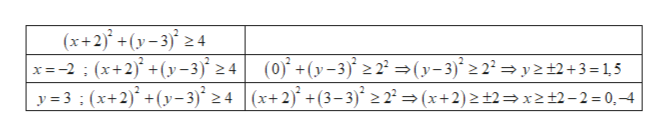Question
2 views

Graph the solution set.

(x + 2)2 + (y − 3)2 ≥ 4
check_circle

Step 1

Here the given equation is (x + 2)2 + (y – 3)2 ≥ 4 equation of circle and (-2, 3) is the center of the circle and the radius ...help_outlineImage Transcriptionclose(x+2)° +(y– 3)° 2 4 x=-2 ; (x+2)° +(y–3)° 2 4 y = 3 ; (x+2)° +(y-3)* 2 4 |(x+2)° +(3– 3)* 2 2ª = (x+2)2±2=x2 +2-2=0,4 (0)° + (y-3)° > 2² =(y-3)* 2 2² = y 2±2+3=1,5 fullscreen

### Want to see the full answer?

See Solution

#### Want to see this answer and more?

Solutions are written by subject experts who are available 24/7. Questions are typically answered within 1 hour.*

See Solution
*Response times may vary by subject and question.
Tagged in

### Other